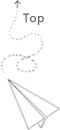# 故障描述：

error日志出现数据库连接异常，而实际交易量似乎并没有到达耗尽连接池的地步。

## 原因分析

```update table1
<set>
<if test="status!= null">
status=#{status},
</if>
<if test="time != null">
time=#{time},
</if>
</set>
where
<if test="name != null">
name=#{name}
</if>
<if test="age != null">
and age=#{age}
</if>
and status="01" or status="02"
```

# 解决方案

```update table1
<set>
<if test="status!= null">
status=#{status},
</if>
<if test="time != null">
time=#{time},
</if>
</set>
where
<if test="name != null">
name=#{name}
</if>
<if test="age != null">
and age=#{age}
</if>
and (status="01" or status="02")
```

## 推荐文章